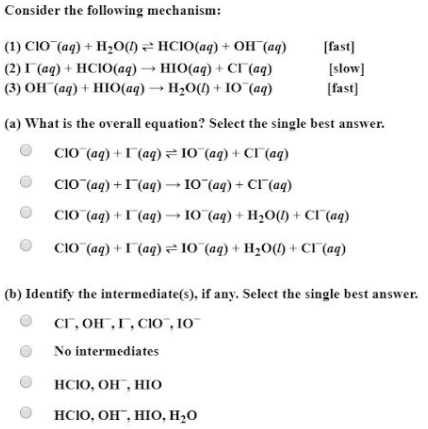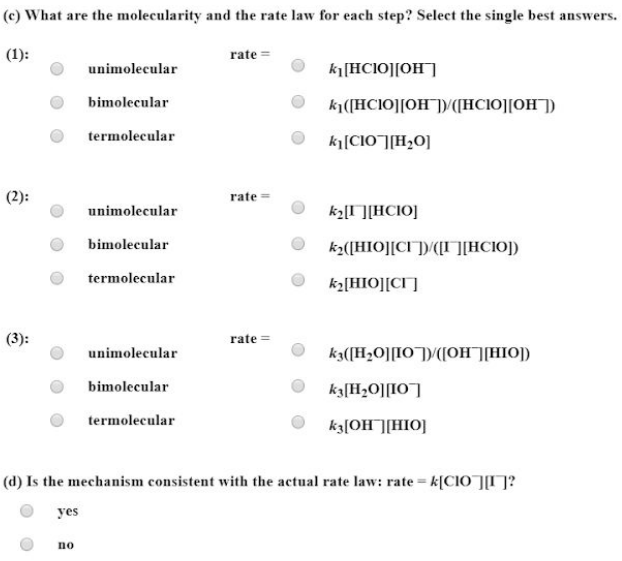# Consider the following mechanism: (1) ClO-(aq) + H2O(l) <-> HClO(aq) + OH-(aq) [fast] (2) I-(aq) + HClO(aq) -> HIO(aq) + Cl-(aq) [slow] (3) OH-(aq) + HIO(aq) -> H2O(l) + IO-(aq) [fast] (a) What is the overall equation? Select the single best answer. (b) Identify the intermediate(a), if any. Select the single best answer. (c) What are the molecularity and the rate law for each step? Select the single best answers. (d) Is the mechanism consistent with the actual rate law: rate = k[ClO-][I-]?